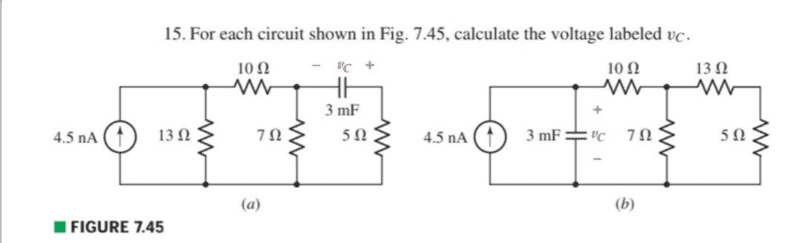# Finding Vc for two circuits, Electric Circuit Analysis question

• Engineering
• Xiao Xiao

#### Xiao Xiao

Homework Statement
For each circuit shown in Fig. 7.45, calculate the voltage labeled vc.
Relevant Equations
In both circuits I should consider an open circuit in place of the capacitor, and then calculate Req for the remaining resistors (5ohm resistor is ommited in circuit-a) then use v=iR to find the the voltage, and by parallel v=vc (capacitor's voltage) is my answer correct? I'm sorry if this is a silly question.I think what you said is correct in case (b), but not in case (a). The capacitor is not in parallel with the current source in case (a). Why don't you try calculating vc in both cases and show us your work?

I think what you said is correct in case (b), but not in case (a). The capacitor is not in parallel with the current source in case (a). Why don't you try calculating vc in both cases and show us your work?
For (b) I did Rth= 15.04ohm, Vc=v=iR=67.68nV

For (a) capacitor is short circuit in dc circuit, so we ignore the 5ohm so Req=7. 37ohm, and v=33.16nV, if what you're saying is correct then I'm not sure how to proceed after that.

#### Attachments

Part (b) looks correct. For part (a), the voltage across the capacitor will be equal to the voltage across the 7 Ohm resistor. How much current is flowing through the 7 Ohm resistor, and what s the voltage across it?

•Xiao Xiao
Part (b) looks correct. For part (a), the voltage across the capacitor will be equal to the voltage across the 7 Ohm resistor. How much current is flowing through the 7 Ohm resistor, and what s the voltage across it?
Oooh, I see, thanks a lot, I'll try to do that now.

In both cases the capacitor is open circuited when the equivalent resistance is calculated as follows. In case (a) you have 17 ohms in parallel with 13 ohms. Collapse that into one resistor and place it in parallel with the open circuited capacitor and just do V=IR. In case (b) you have 18 ohms in parallel with 7 ohms and then in series with 10 ohms. Collapse that into one resistor in parallel with the open circuited capacitor and, once again, do V=IR.

•Xiao Xiao